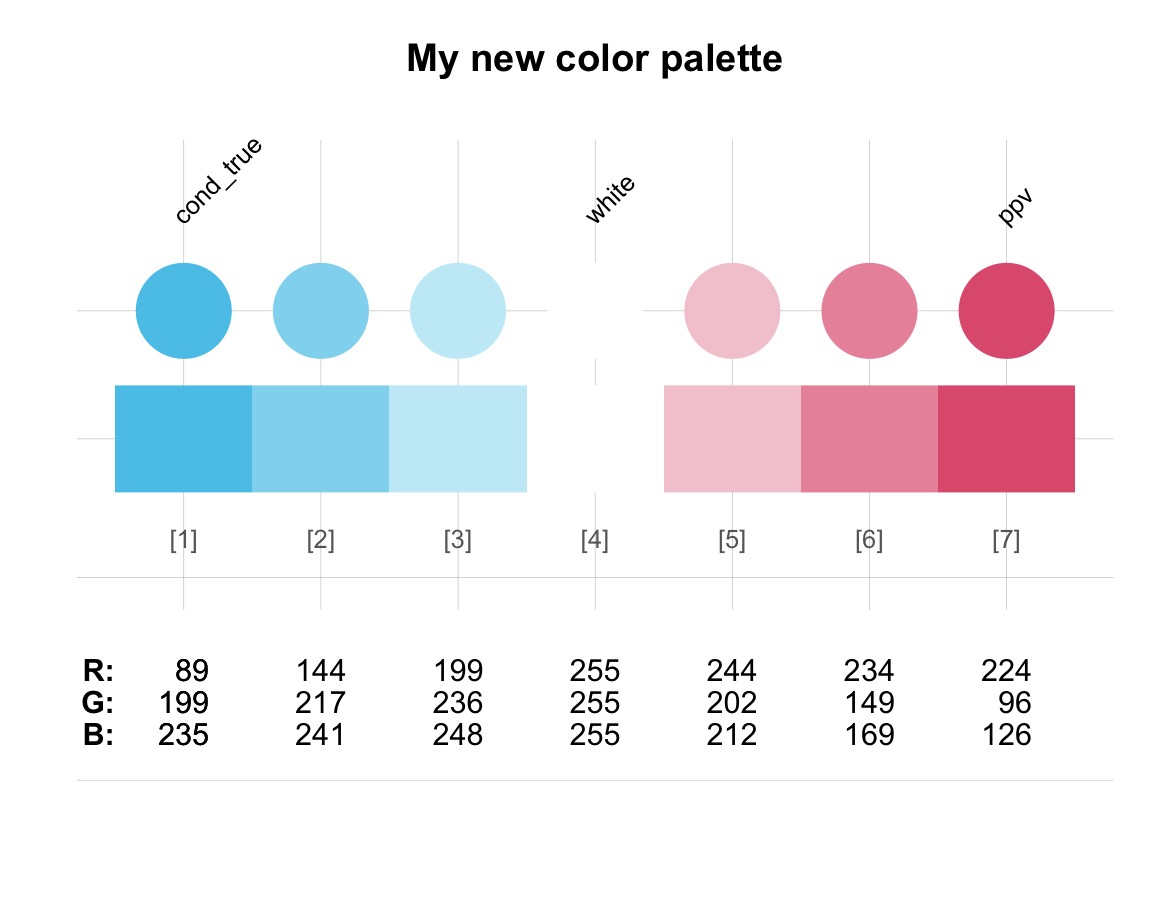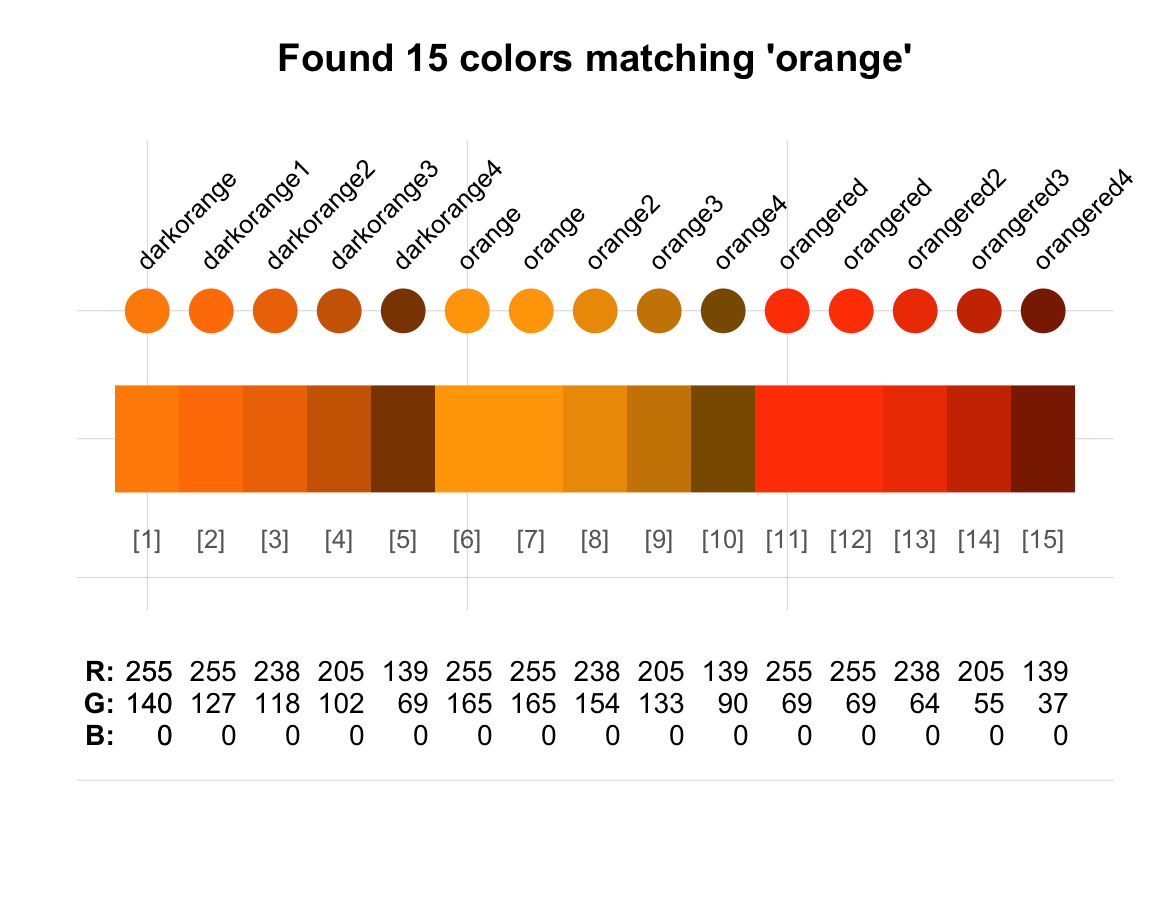## 6.2 The pipe from magrittr

The dplyr R package is awesome.
Pipes from the magrittr R package are awesome.
Put the two together and you have one of the most exciting things to happen to R in a long time.

Sean C. Anderson (2014)

The pipe operator from the magrittr package is a simple tool for data manipulation. Essentially, the so-called pipe operator %>% allows chaining commands in which the current result is passed to the next command (from left to right). With such forward pipes, a sequence of simple commands can be chained into a powerful compound command. This will be particularly useful when transforming tables with dplyr (in Section 6.3) and tidyr (in Section 6.4). However, the following section shows that the pipe is an interesting tool in itself.

### 6.2.1 The function of pipesFigure 6.1: Ceci est un pipe: %>%.

What is a pipe? In the subdiscipline of physics known as plumbing, a pipe is a device for directing fluid and solid substances from one location to another. In R, the pipe is an operator that allows re-writing multiple functions as a chain of individual functions. For our present purposes, it is sufficient to think of the pipe operator %>% as passing whatever is on its left to the first argument of the function on its right. For instance, given three functions a(), b() and c(), the following pipe would compute the result of the compound expression c(b(a(x))):

# Apply a to x, then b, then c:
x %>% a() %>% b() %>% c()

# typically written as:
x %>%
a() %>%
b() %>%
c()

To assigning the result of a pipe to some object y, we need to use the assignment operator at the (top) left of the pipe:

# Apply a to x, then b, then c
# and assign the result to y:
y <- x %>% a() %>% b() %>% c()

# typically written as:
y <- x %>%
a() %>%
b() %>%
c()

### 6.2.2 Example pipes

We will mostly use pipes for manipulating tables in R. However, the following examples illustrate that pipes can be used for other tasks as well.

#### Arithmetic pipes

Whereas a description of the pipe operator may sound complicated, the underlying idea is quite simple: We often want to perform several operations in a row. This is familiar in arithmetic expressions. For instance, consider the following step-by-step instruction:

• Start with a number x (e.g., x = 3). Then,
• multiply it by 4,
• add 20 to the result,
• subtract~7 from the result, and finally
• take the result’s square root.

This instruction can easily be translated into the following R expression:

x <- 3
sqrt((x * 4) + 20 - 7)
#>  5

In this expression, the order of operations is determined by parentheses, arithmetic rules (e.g., left to right, multiplying before adding and substracting, etc.), and functions. Avoiding the infix operators * and +, we can re-write the expression as a sequence of R functions:

sqrt(sum(prod(x, 4), 20, -7))
#>  5

The order of function application is determined by their level of encapsulation in parentheses. The pipe operator %>% allows us re-writing the sequence of functions as a chain:

x %>% prod(4) %>% sum(20, -7) %>% sqrt()
#>  5

Note that this pipe is fairly close to the step-by-step instruction above, particularly when we re-format the pipe to span multiple lines:

x %>%
prod(4) %>%
sum(20, -7) %>%
sqrt()
#>  5

Thus, the pipe operator lets us express chains of function applications in a way that matches their natural language description.

If we find the lack of an explicit representation of each step’s result on the right hand side of %>% confusing, we can re-write the piped command as follows:

x %>% prod(., 4) %>% sum(., 20, -7) %>% sqrt(.)
#>  5

Here, the dot . represents whatever was passed (or “piped”) from the left to the right (here: the current value of x).

While the pipe initially may seem somewhat similar to our assignment operator <-, they are actually quite different. For instance, the pipe does not assign new objects, but rather apply functions to an existing objects that serves as the input. The input object changes as functions are being applied and eventually result in an output object. Assuming there is no function y(), the following code would not assign anything to y, but yield an error:

x %>%
prod(4) %>%
sum(20, -7) %>%
sqrt() %>%
y

Thus, for assigning the result of a pipe to an object y, we need to use our standard assignment function on the left (or at the beginning) of the pipe:

y <- x %>%
prod(4) %>%
sum(20, -7) %>%
sqrt()
y
#>  5

Overall, the pipe operator %>% does not allow us to do anything we could not do before, but allows us re-writing chains of commands in a more natural fashion. Essentially, embedded calls of functions within functions are untangled into a linear chain of steps. This is particularly useful when generating and transforming data objects (e.g., vectors or tables) by a series of functions that all share the same type of inputs and outputs (e.g., vectors or tables). Importantly, using the pipe makes complex sequences of function calls easier to construct and understand.

A key requirement for using the pipe is that we are aware of the data structures serving as inputs and outputs at each step. Additionally, piping functions implies that we do not need the results of intermediate steps.

#### Color pipes

Using the pipe operator %>% requires that functions accept some key input as their first argument. Fortunately, most R functions are written in just this way. As a concrete and colorful example, consider the usecol() and seecol() functions of the unikn package :

library(unikn)

Besides defining some custom colors (like Seeblau or Pinky), the unikn package provides two general functions for creating and viewing color palettes:

• The usecol() function uses an input argument pal to define a color palette (e.g., as a vector of color names) and extends this palette to n values. Its output is a color palette (as a vector of color codes).

• The seecol() function shows and provides detail information on a given color palette.

A typical task when selecting colors is to define a new color palette and then visually inspecting them. As the first input argument of seecol() matches the output of usecol(), we can use the pipe operator to chain both commands:

usecol(c(Seeblau, "white", Pinky), n = 7) %>%
seecol(title = "My new color palette")A more traditional (and explicit) version of the same commands would first use usecol() for defining a color palette as an R object (e.g., my_col) and then use this as the first argument of the seecol() function:

my_col <- usecol(c(Seeblau, "white", Pinky), n = 7)
seecol(pal = my_col, title = "My new color palette")

Note that both of these code snippets call the same functions and create the same visualization. However, using the pipe did not define my_col as a separate object in our environment. Thus, the piped chain solution is more compact and immediate, but the second solution additionally allows us to use my_col later.

#### Practice

The grepal() function of unikn searches all R color names (in colors()) for some term (e.g., “gold,” “orange,” or “white”) and returns the corresponding colors.

• Construct some color pipes that finds different versions of key colors and displays the corresponding colors.

• Are there more “black” or more “white” colors in R?

Solution:

A pipe that shows all “orange” colors (i.e., colors with “orange” in their name) would be:

grepal("orange") %>% seecol(title = "Shades of 'orange' in R")There is only one black, but many different types of “white” in R:

grepal("black") %>% seecol()
grepal("white") %>% seecol()

# Note:
grepal("grey")  %>% seecol()
grepal("dark")  %>% seecol()
grepal("light") %>% seecol()

Overall, these examples show that the pipe operator %>% of the magrittr package facilitates writing R expressions in many contexts.

#### Transition

As we have seen, the pipe can be used to feed data inputs into functions, but is particularly useful when modifying tables of data (i.e., data frames or tibbles). In the two main sections of this chapter, we will be using pipes to illustrate the tools provided by two popular tidyverse packages:

• Section 6.3: Transforming tables with dplyr

• Section 6.4: Transforming tables with tidyr

A good question to ask at this point would be: If both these packages transform data tables, what is the difference between dplyr and tidyr? We will address this question after introducing the essential commands of both packages (in Section 6.5.1).

### References

Bache, S. M., & Wickham, H. (2014). magrittr: A forward-pipe operator for R. https://CRAN.R-project.org/package=magrittr
Neth, H., & Gradwohl, N. (2021). unikn: Graphical elements of the university of konstanz’s corporate design. https://CRAN.R-project.org/package=unikn
Wickham, H., François, R., Henry, L., & Müller, K. (2021). dplyr: A grammar of data manipulation. https://CRAN.R-project.org/package=dplyr
Wickham, H., & Henry, L. (2020). tidyr: Tidy messy data. https://CRAN.R-project.org/package=tidyr